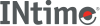﻿ fmodINtime SDK Help
fmod

Calculates the floating-point remainder.

```#include <math.h>

double fmod (double x, double y);
```

#### Parameters

`x, y`
Floating-point values.

#### Remarks

Calculates `f` of `x`/`y` such that:

```x = i * y + f
```

Where:

`i`
An integer.
`f`
The floating-point remainder. `f` has the same sign as `x`, and the absolute value of `f` is less than the absolute value of `y`.

#### Return Values

The remainder.
Success.
0 (zero) if `y` is 0.
Failure.

This function does not return standard ANSI domain or range errors.

#### Requirements

Versions Defined in Include Link to
INtime 3.0 intime/rt/include/math.h math.h clib.lib